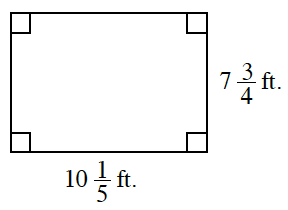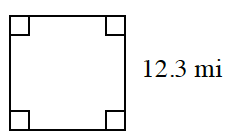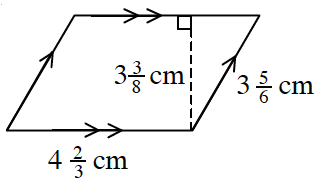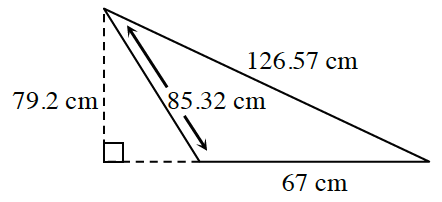Home > CC2MN > Chapter cc26 > Lesson cc26.2.4 > Problem6-100

6-100.

Find the area and perimeter of the following figures. Homework Help ✎

1.Remember, the perimeter is the sum of all sides while the area is the value of the space within the perimeter.

$\text{Area}=(\text{base})(\text{height})$
$\text{Perimeter}\ \text{of a rectangle}=2(\text{base})+2(\text{height})$

$A=79\frac{1}{20}\text{f}t^{2}$

$P=35\frac{9}{10}\text{ft}$

1.Remember, a square has $4$ sides of equal lengths.

1.The area of a parallelogram is found by multiplying the base by the height. The perimeter is the sum of the $4$ sides.

1.The area of a triangle is found by multiplying the base by the height and dividing that product by $2$.

$A=2653.2$ cm2
$P=278.89$ cm